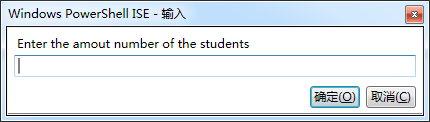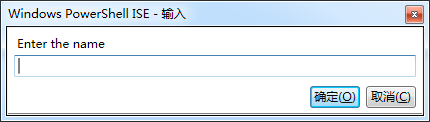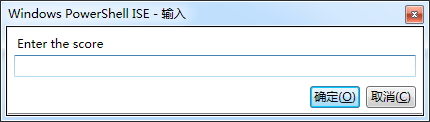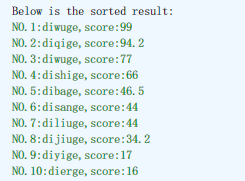#0
0
01. 云栖社区>
2. 博客>
3. 正文

## 冒泡排序——PowerShell版```\$count = Read-Host "Enter the amout number of the students"
\$studentName = New-Object System.Collections.ArrayList
\$studentScore = New-Object System.Collections.ArrayList
for(\$i=1;\$i -le [int]\$count;\$i++)
{
\$name = Read-Host "Enter the name"
\$score= Read-Host "Enter the score"
}
#Sort begin.
for(\$i=1;\$i -le \$count-1;\$i++)
{
for(\$j=1;\$j -le \$count-\$i;\$j++)
{
#Compare the students' values.
if([int]\$studentScore[\$j-1] -le [int]\$studentScore[\$j])
{
#Exchange the score.
\$s = \$studentScore[\$j-1]
\$studentScore[\$j-1] = \$studentScore[\$j]
\$studentScore[\$j] = \$s
#Exchange the name.
\$n = \$studentName[\$j-1]
\$studentName[\$j-1] = \$studentName[\$j]
\$studentName[\$j] = \$n
}
}
\$i++
}
#Print the sorted result.
Write-Host "Below is the sorted result:"
for(\$i=0;\$i -le \$count-1;\$i++)
{
\$j=\$i+1
\$tip = "NO."+\$j+":"+\$studentName[\$i]+",score:"+\$studentScore[\$i]
Write-Host \$tip -ForegroundColor Green
}```

1、外for循环代表进行\$count-1轮排序；

2、内for循环代表从第一轮排序开始，第“\$i”轮排序将进行\$count-\$i次比较。+ 关注